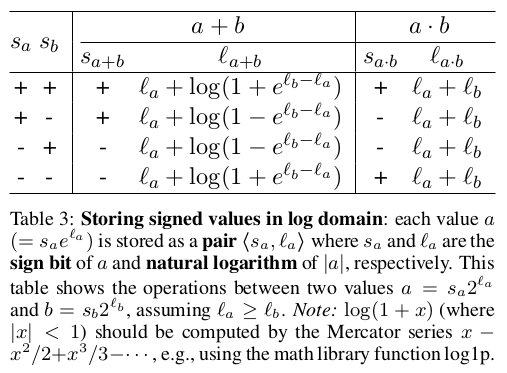# Log-Real number class

Most people know how to avoid numerical underflow in probability computations by representing intermediate quantities in the log-domain. This trick turns "multiplication" into "addition", "addition" into "logsumexp", "0" into $$-\infty$$ and "1" into $$0$$. Most importantly, it turns really small numbers into reasonable-size numbers.

Unfortunately, without modification, this trick is limited to positive numbers because log of a negative number is NaN.

Well, there is good news! For the cost of an extra bit, we can extend this trick to the negative reals and furthermore, we get a bonafide ring instead of a mere semiring.

I first saw this trick in Li and Eisner (2009). The trick is nicely summarized in Table 3 of that paper, which I've pasted below.Why do I care? When computing gradients (e.g., gradient of risk), intermediate values are rarely all positive. Furthermore, we're often multiplying small things together. I've recently found log-reals to be effective at squeaking a bit more numerical accuracy.

This trick is useful for almost all backprop computations because backprop is essentially:

adjoint(u) += adjoint(v) * dv/du.


The only tricky bit is lifting all du/dv computations into the log-reals.

Implementation:

• This trick is better suited to programming languages with structs. Using objects will probably in an horrible slow down and using parallel arrays to store the sign bit and double is probably too tedious and error prone. (Sorry java folks.)

• Note that log-real += involves calls to log and exp, which will definitely slow your code down a bit (these functions are much slower than addition).